## Selina Concise Mathematics Class 10 ICSE Solutions Chapter 5 Quadratic Equations Ex 5C

These Solutions are part of Selina Concise Mathematics Class 10 ICSE Solutions. Here we have given Selina Concise Mathematics Class 10 ICSE Solutions Chapter 5 Quadratic Equations Ex 5C.

Other Exercises

Question 1.
Solve each of the following equations, using the formula:
Solution: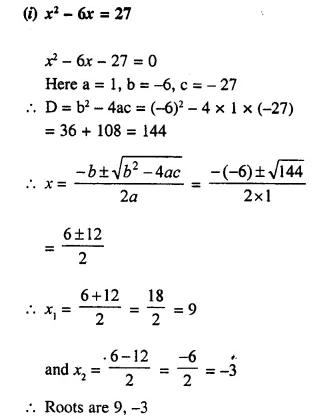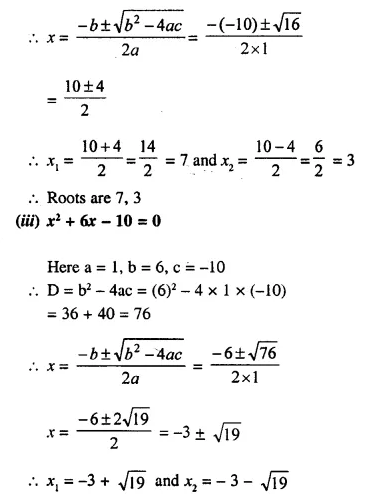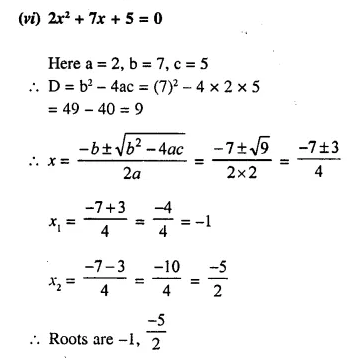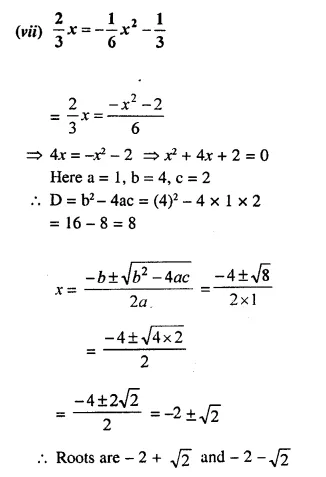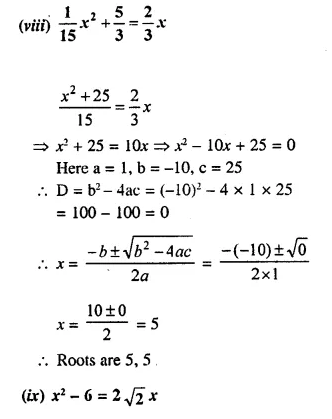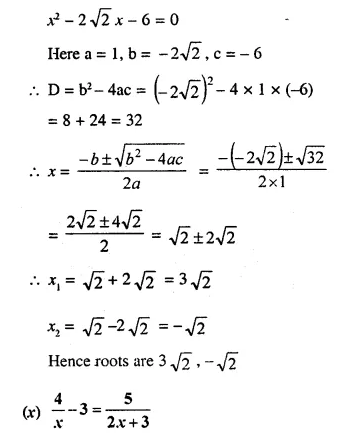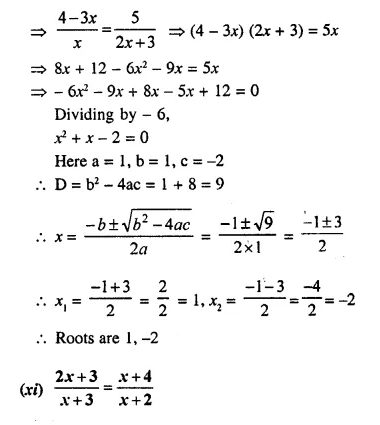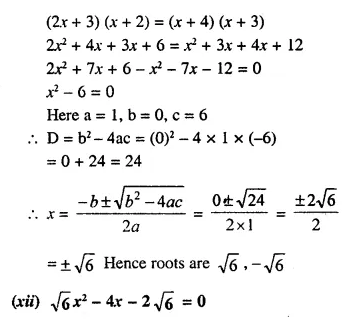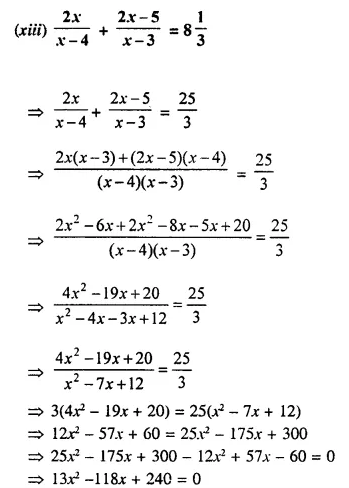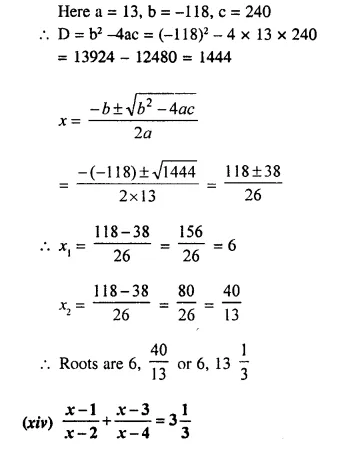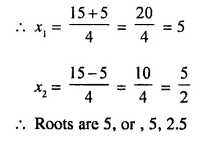Question 2.
Solve each of the following equations for x and give, in each case, your answer correct to one decimal place :
(i) x² – 8x + 5 = 0
(ii) 5x² + 10x – 3 = 0
Solution: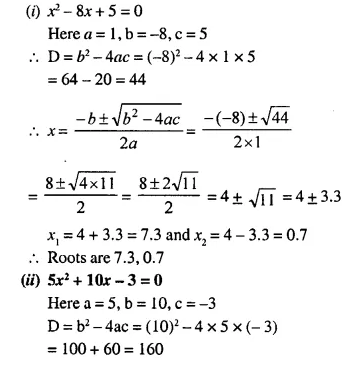Question 3.
Solve each of the following equations for x and give, in each case, your answer correct to 2 decimal places :
(i) 2x² – 10x + 5 = 0
(ii) 4x² + $$\frac { 6 }{ x }$$ + 13 = 0
(iii) x² – 3x – 9 = 0 
(iv) x² – 5x – 10 = 0 
Solution: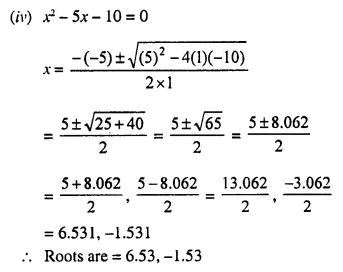Question 4.
Solve each of the following equations for x, giving your answer correct to 3 decimal places:
(i) 3x² – 12x – 1 = 0
(ii) x² – 16x + 6 = 0
(iii) 2x² + 11x + 4 = 0
Solution: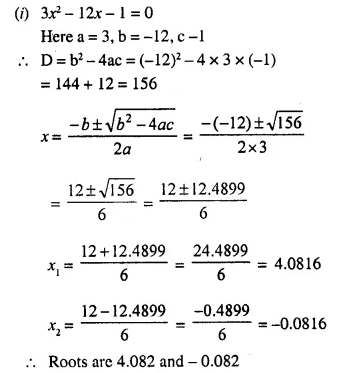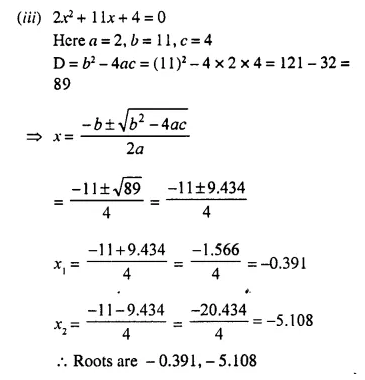Question 5.
Solve:
(i) x4 – 2x² – 3 = 0
(ii) x4 – 10x² + 9 = 0
Solution:Question 6.
Solve:
(i) (x² – x)² + 5 (x² – x) + 4 = 0
(ii) (x² – 3x)² – 16 (x² – 3x) – 36 = 0
Solution: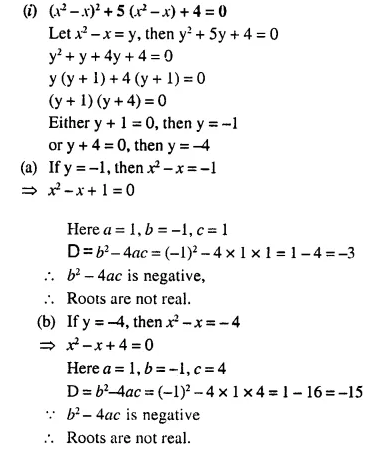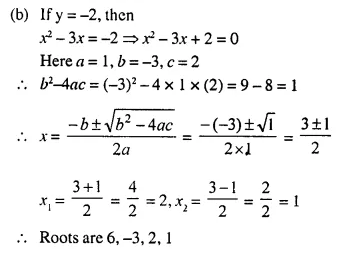Question 7.
Solve :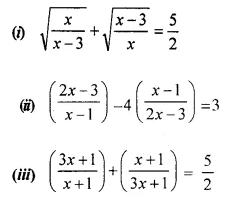Solution: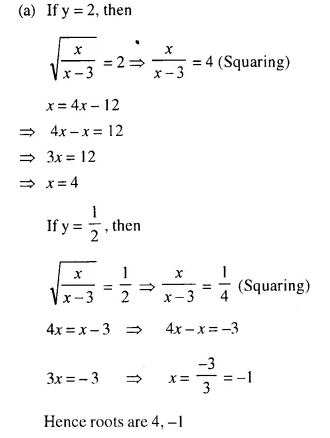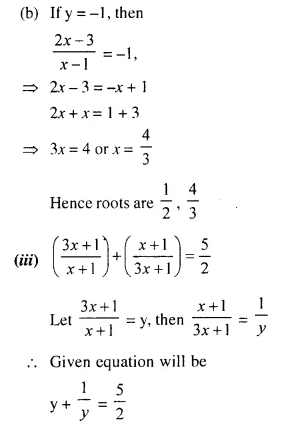Question 8.
Solve the equation : 2x – $$\frac { 1 }{ x }$$ = 7. Write your answer correct to two decimal places.
Solution: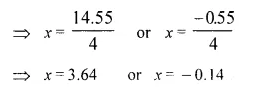Question 9.
Solve the following equation and give your answer correct to 3 significant figures : 5x² – 3x – 4 = 0
Solution:Question 10.
Solve for x using the quadratic formula. Write your answer correct to two significant figures, (x – 1)² – 3x + 4 = 0
Solution: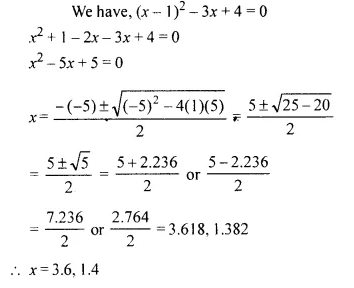Question 11.
Solve the quadratic equation x² – 3 (x + 3) = 0; Give your answer correct to two significant figures.
Solution: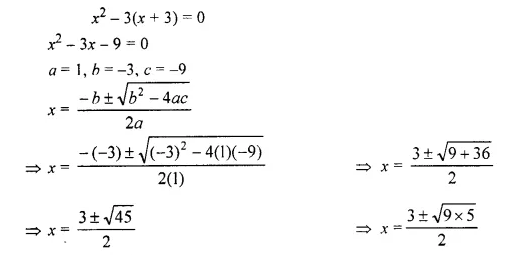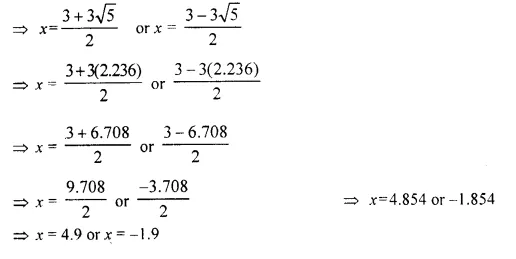Hope given Selina Concise Mathematics Class 10 ICSE Solutions Chapter 5 Quadratic Equations Ex 5C are helpful to complete your math homework.

If you have any doubts, please comment below. Learn Insta try to provide online math tutoring for you.